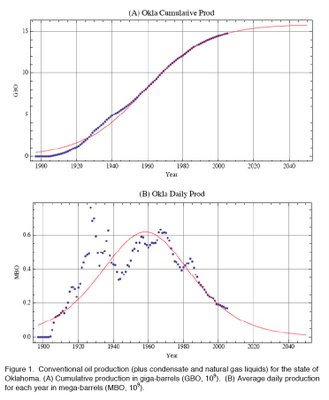Thursday, January 15, 2009

Exact Verhulst Solution

Date: Thu, 8 Jan 2009 14:54:12 -0700 (MST)
From: Willy Hereman
To: John Stockwell
Cc: Doug Baldwin
Subject: Re: Chris Liner's paper using the Verhulst eq

John and Doug,

I read Liner's article (attached for you Doug).  Interesting!  I also did some work on the Verhulst equation, i.e. eq. (1) in his paper:

q'(t) = a_1 q - (a_1/a_2) q^2,

where a_1 is the intrinsic growth rate and a_2 is the saturation level
(also called the carrying capacity).

Eq. (1) has an exact solution which can be computed by separation of variables or by treating (1) as a Bernoulli equation. The solution is then represented as a rational expression involving an exponential function. That form of the solution can be found in almost any book on ODEs.

However, by looking at Liner's curve of the derivative, q'(t), in Fig. 1 (b), it came to me that the exact solution might be expressible in terms of a tanh function for its derivative is then sech-squared (a bell shaped, not to be confused with a true Gaussian curve, although they look alike).

Several years ago, Douglas Baldwin and I desigend a Mathematica program that automatically computes the exact tanh solutions of ODE and PDEs.  So, I tried our program and here is the nice closed form solution of Eq. (1) produced by the code:

q(t) = (1/2) a_2 { 1 + tanh[ (1/2) a_1 t + delta ] }

and its derivative

q'(t) = (1/4) a_1 a_2 sech^2 [ (1/2) a_1 t + delta ]

These are the exact mathematical expressions of the curves Liner
plotted in Fig. 1 (a) and (b), respectively.

Well, I learned something today.  I had not realized up to now that Verhulst's logistic equation had a simple tanh solution!

I have attached the Mathematica notebook with the result obtained
by our PDESpecialSolutionsV2.m code (the code is also attached).

Best,

Willy

ps (5-apr-2011)

The delta in the solution is an arbitrary constant. It is equivalent to writing the solution as

q(t) = (1/2) a_2 { 1 + tanh[ (1/2) a_1 (t - t_0) ] }

for an arbitrary t_0, i.e., an initial value for time t. The remaining two constants are a_1 and a_2.

Willy

------------

Dr. Willy A. Hereman, Professor
Department of Mathematical and Computer Sciences